# 《算法》笔记 10 - 无向图

• 表示无向图的数据结构
• 邻接表数组
• 深度优先搜索
• 深度优先搜索寻找路径
• 深度优先搜索的性能特点
• 广度优先搜索
• 两种搜索方式的对比

### 表示无向图的数据结构

 public class Graph{
Graph(int V)   //创建一个含有V个顶点但不含有边的图
Graph(In in)   //从标准输入流in读入一幅图
int v()     //顶点数
int E()    //边数
void addEdge(int v， int w)    //向图中添加一条边v-w
String toString()      //对象的字符串表示
}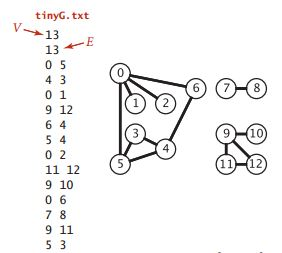#### 邻接表数组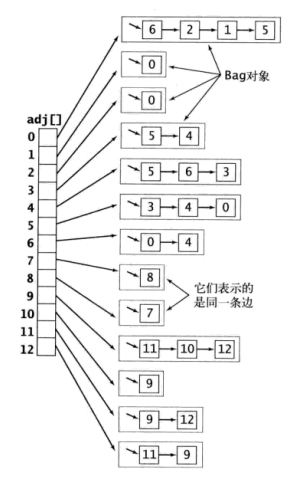public class Graph {
private final int V; // vertex
private int E; // edge

public Graph(int V) {
this.V = V;
this.E = 0;
for (int v = 0; v < V; v++) {
}
}

public Graph(In in) {
for (int i = 0; i < E; i++) {
}
}

public int V() {
return V;
}

public int E() {
return E;
}

public void addEdge(int v, int w) {
E++;
}

}
}

• 使用的空间和V+E成正比
• 添加一条边所需的时间为常数
• 遍历顶点一个顶点的相邻顶点所需的时间和这个顶点的度数成正比（顶点的度数表示与这个顶点相连的边数）

### 深度优先搜索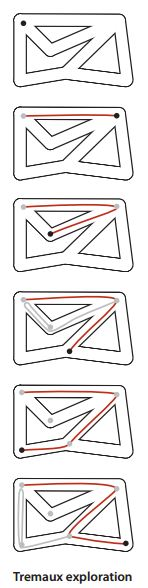public class DepthFirstSearch {
private boolean[] marked;
private int count;
private final int s;

public DepthFirstSearch(Graph G, int s) {
marked = new boolean[G.V()];
this.s = s;
dfs(G, s);
}

private void dfs(Graph G, int v) {
marked[v] = true;
for (int w : G.adj(v)) {
if (!marked[w]) {
dfs(G, w);
}
}
}

public boolean marked(int w) {
return marked[w];
}

public int count() {
return count;
}
}

#### 深度优先搜索寻找路径

public class DepthFirstPaths {
private boolean[] marked;
private int[] edgeTo;  //新增的，用于记录路径
private final int s;

public DepthFirstPaths(Graph G, int s) {
marked = new boolean[G.V()];
edgeTo = new int[G.V()];
this.s = s;
dfs(G, s);
}

private void dfs(Graph G, int v) {
marked[v] = true;
for (int w : G.adj(v)) {
if (!marked[w]) {
edgeTo[w] = v;  //记录路径
dfs(G, w);
}
}
}

public boolean marked(int w) {
return marked[w];
}

public int count() {
return count;
}

public boolean hasPathTo(int v) {   //判断是否存在从s到v的路径
return marked(v);
}

public Iterable<Integer> pathTo(int v) {  //获取从s到v的路径，不存在则返回null
if (!hasPathTo(v))
return null;

Stack<Integer> path = new Stack<Integer>();
for (int x = v; x != s; x = edgeTo[x]) {
path.push(x);
}

path.push(s);
return path;
}

public static void main(String[] args) {
In in = new In(args);
Graph G = new Graph(in);
int s = Integer.parseInt(args);
DepthFirstPaths search = new DepthFirstPaths(G, s);

//
for (int v = 0; v < G.V(); v++) {
StdOut.print(s+" to "+v+": ");
if(search.hasPathTo(v)){
for(int x:search.pathTo(v)){
if(x==s) StdOut.print(x);
else StdOut.print("-"+x);
}
}
StdOut.println();
}
}
}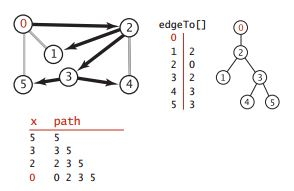java DepthFirstPaths tinyCG.txt 0
0 to 0：0
0 to 1：0-2-1
0 to 2：0-2
0 to 3：0-2-3
0 to 4：0-2-3-4
0 to 5：0-2-3-5

### 广度优先搜索

public class BreadthFirstPaths {
private boolean[] marked;
private int[] edgeTo;
private final int s;

public BreadthFirstPaths(Graph G, int s) {
marked = new boolean[G.V()];
edgeTo = new int[G.V()];
this.s = s;
bfs(G, s);
}

private void bfs(Graph G, int s) {
Queue<Integer> queue = new Queue<Integer>();
marked[s] = true;
queue.enqueue(s);
while (!queue.isEmpty()) {
int v = queue.dequeue();
for (int w : G.adj(v)) {
if(!marked[w]){
edgeTo[w]=v;
marked[w]=true;
queue.enqueue(w);
}
}
}
}

public boolean hasPathTo(int v){
return marked[v];
}

public Iterable<Integer> pathTo(int v) {
if (!hasPathTo(v))
return null;

Stack<Integer> path = new Stack<Integer>();
for (int a = v; a != s; a = edgeTo[a]) {
path.push(a);
}

path.push(s);
return path;
}

// cmd /c --% java algs4.four.BreadthFirstPaths ..\..\..\algs4-data\tinyCG.txt 0
public static void main(String[] args) {
In in = new In(args);
int s = Integer.parseInt(args);
Graph g = new Graph(in);

for (int i = 0; i < g.V(); i++) {
StdOut.print(i + ":");
Iterable<Integer> path = search.pathTo(i);
for (Integer p : path) {
if (search.s != p) {
StdOut.print("-" + p);
} else {
StdOut.print(p);
}
}
StdOut.println();
}
}
}

• 取队列中的下一个顶点v并标记它
• 将与v相邻的所有未被标记过的顶点加入队列。
队列先进先出(FIFO)的特性可以达到广度优先搜索寻找距离逐渐增大的效果。在深度优先搜索中，实际上隐式地使用了一个遵循后进先出(LIFO)规则的栈，在dfs的递归调用的过程中，这个栈由系统管理。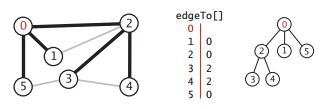### 两种搜索方式的对比

• 取其中的下一个顶点v并标记它
• 将与v相邻而又未被标记过的顶点加入数据结构中
两种算法的区别在于从数据结构中获取下一个顶点的规则，深度优先搜索会首先取最晚加入数据结构的顶点，而广度优先搜索取得则是最早加入的顶点。这种规则的区别会影响搜索图的路径，深度优先搜索会不断深入图中，并在栈中保存了所有分叉的顶点，广度优先搜索则像扇面一般扫描图，用一个队列保存访问过的最前段的顶点。
posted @ 2019-11-24 21:08  zhixin9001  阅读(...)  评论(...编辑  收藏